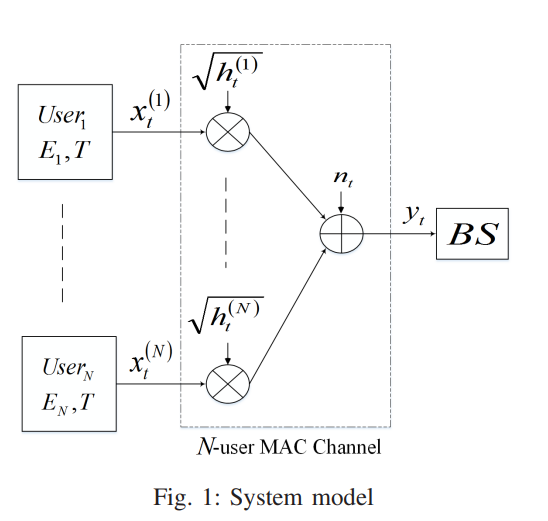Conference Paper

# Optimal energy allocation for delay-constrained traffic over fading multiple access channels

By
Girgis A.M.
El-Keyi A.
Nafie M.

In this paper, we consider a multiple-access fading channel where N users transmit to a single base station (BS) within a limited number of time slots. We assume that each user has a fixed amount of energy available to be consumed over the transmission window. We derive the optimal energy allocation policy for each user that maximizes the total system throughput under two different assumptions on the channel state information. First, we consider the offline allocation problem where the channel states are known a priori before transmission. We solve a convex optimization problem to maximize the sum-throughput under energy and delay constraints. Next, we consider the online allocation problem, where the channels are causally known to the BS and obtain the optimal energy allocation via dynamic programming when the number of users is small. We also develop a suboptimal resource allocation algorithm whose performance is close to the optimal one. Numerical results are presented showing the superiority of the proposed algorithms over baseline algorithms in various scenarios. © 2016 IEEE.